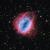# OctahedronOctahedron is the third platonic solid. It consists of 8 faces (each is an equilateral triangle) and 6 vertices (each at the meeting of 4 faces).

## Creation

There are multiple methods for creating an octahedron, depending upon how you look at the solid.

• Looking at the edges,
• Looking at the faces – trigonometry approach
• Duality - From a hexahedron (cube)

### Looking at edges

An octahedron can be considered as 3 squares intersecting at corners.

• create the first square

As each vertex is the meeting of 4 edges, we need to connect 2 edges to each of the existing vertices.

• The next square intersects two opposing corners of our existing square, hence we need to change our orientation to face the opposing corner LT 45
• Our second square also needs to be vertical, rather than horizontal, hence, our code changes from RT 90 to RD 90.
• Finally, before we can start, we need to change our orientation to be pointing up 45 degrees to be heading in the correct direction for the first edge, this is accomplished with the instruction RU 45

This same routine needs to be repeated for the second pair of opposing corners.

Note: these instructions are only appropriate for creating the outline of an octahedron, to fill in the faces additional instructions are required.

### Trigonometry

• We know that an octahedron is made from equilateral triangles, hence, we start with a single equilateral triangle.
• We now need to determine the dihedral angle (angle between faces)

If we consider the octahedron as two square based pyramids joined at the bases, we can use right angle trigonometry to determine the dihedral angle – first by finding the angle between the square base and each triangular face, then doubling this answer (for when the two pyramids are joined)

• Using this angle, we are now able to create the next 3 faces (connected to our original triangle)

The command RT 60 orientates the turtle to look back along the last triangle edge created, we the use the TR command to create the dihedral angle

this process now gives the 4 triangles which form the 'top' of our octahedron. At the base of each of the for triangles, we can now create 4 new triangles, once again utilising the dihedral angle, the new command now reads

### Duality

Platonic Solids are consider to have duality

Every polyhedron has a dual (or "polar") polyhedron with faces and vertices interchanged. The dual of every Platonic solid is another Platonic solid, so that we can arrange the five solids into dual pairs.

The hexahedron and octahedron are duals, this means that if we take the midpoint of each face of an octahedron, connecting the points will create a hexahedron and vice versa.

Starting with our cube, we can see that there are 6 points A-F found at the centre of each face. these 6 points become the vertices of our octahedron, connecting A-B, A-C, A-D, A-E, F-B, F-C, F-D, F-E, B-C, C-D, D-E, E-B

### Resources

http://en.wikipedia.org/wiki/Platonic_solid

http://en.wikipedia.org/wiki/Octahedron

regular_octohedron.logo (outline only)

octahedron_from_trigonometry.logo (outline only)

octahedron_incube.logo (faces shown)